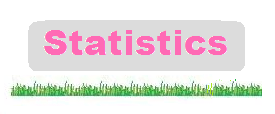### Scatter Diagrams and Line of Best Fit

After plotting the scatter diagram, a line of best fit can be drawn through the data

Use the line of best fit to estimate the value of one variable when the other is given.

#### Example 1.   (a)   Draw the line of best fit(b)   Estimate the weight when the height is 8 metres.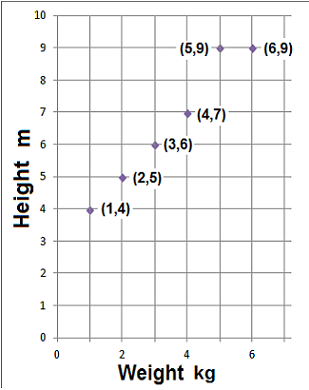(a) The line of best fit is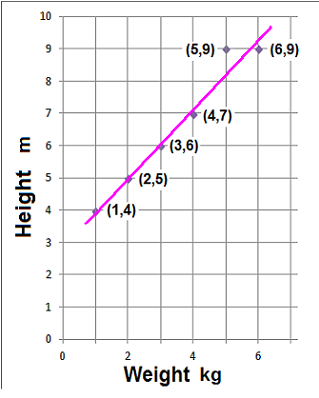(b) Draw a line across where the weight is 8 metres, and then when it hits the line of best fit, draw a line down to estimate the weight.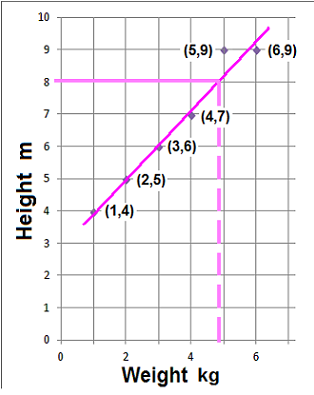The weight is about 4.8 kg

#### Example 2.   (a)   Draw the line of best fit(b)   Estimate the value of the car when it is 6 years old.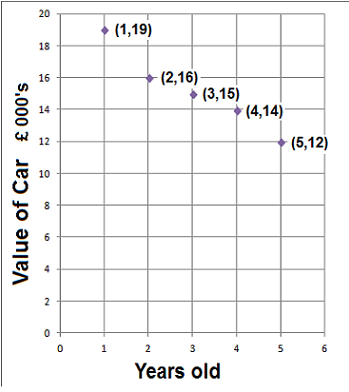(a) The line of best fit is(b) From year 6 draw a line up to the line of best fit. Then from the line of best fit draw a line across to estimate the value of the car.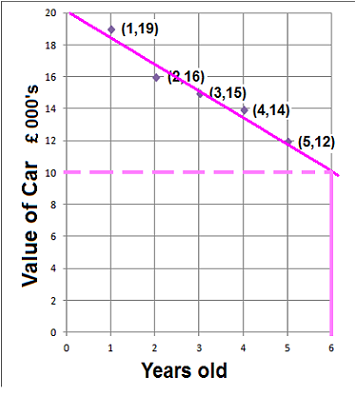The value of the car is around £10,000to: